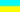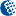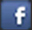Alter.Org.UA<< BackHomeUKDonate### Implementation of CommandLineToArgv for porting console apps from Unix to Win32

#### by Boris Timofeev aka boristim_at_mail.ru

I often use parts of GPL code in my own software. I've written the following function to minimize code changes wnen porting apps. It is enough to replace "main" function with "XXXX_main" and prepare argc/argc with my CommandLineToArgvAEx() if the application doesn't expect STDIN. Since argv should contain path to executable we have to either prepend path to commangline or just reserve (and keep empty) argv.

This implementation allows parsing commandline-like strings in arbitrary encodings.

Find the sources of CommandLineToArgvAEx() and related functions below:

#### cmdl2argv.c

```#include

char *utf16_to_char(LPWSTR str, int cp, int *plength)
{
char *p = NULL;
int cc;
// get length (cc) of the new multibyte string excluding the \0 terminator first
if (plength)
*plength = 0;
if ((cc = WideCharToMultiByte(cp, 0, str, -1, NULL, 0, 0, 0) - 1) > 0)
{	// convert
if (NULL != ( p = (char *)LocalAlloc(LMEM_FIXED, (cc + 1) * sizeof(char)))) {
WideCharToMultiByte(cp, 0, str, -1, p, cc + 1, 0, 0);
if (plength)
*plength = cc;
}
}
return p;
}

wchar_t *char_to_utf16(const char *str, int cp, int *plength)
{
wchar_t *p=NULL;
int cc = 0;
if (plength)
*plength = 0;
// get length (cc) of the new widechar excluding the \0 terminator first
if ((cc = MultiByteToWideChar(cp, 0, str, -1, NULL, 0) - 1) > 0) {	// convert
if (NULL != (p = (wchar_t *)LocalAlloc(LMEM_FIXED, (cc+1)*sizeof(wchar_t)))) {
MultiByteToWideChar(cp, 0, str, -1, p, cc + 1);
if (plength)
*plength = cc;
}
}
return p;
}

char **CommandLineToArgvAEx(char *cmdline, int *p_my_args, int reserv, int src_cp, int dst_cp )
{
char **argv = NULL, **scattered_argv;
*p_my_args = 0;
LPWSTR *args;
size_t count_of_mem = 0;
int cc;
wchar_t *s = char_to_utf16(cmdline, src_cp, NULL);
if (NULL != s) {
if (NULL != (args = CommandLineToArgvW(s, p_my_args))) {
LocalFree(s);
if (NULL != (scattered_argv = (char **)LocalAlloc(LMEM_FIXED, *p_my_args * sizeof(char *)))) {
for (int i = 0; i < *p_my_args; ++i)
if (NULL != (scattered_argv[i] = utf16_to_char(args[i], dst_cp, &cc)))
count_of_mem += (cc + 1)*sizeof(char);
if (count_of_mem) {
count_of_mem += (*p_my_args + reserv) * sizeof(char *);
if (NULL != (argv = (char **)LocalAlloc(LMEM_FIXED|LMEM_ZEROINIT, count_of_mem))) {
cc = (*p_my_args + reserv) * sizeof(char *);
for (int i = reserv; i < *p_my_args + reserv; ++i) {
if (NULL != scattered_argv[i - reserv]) {
argv[i] = (char *)argv + cc;
strcpy(argv[i], scattered_argv[i - reserv]);
cc += (int)strlen(argv[i]) + 1;
LocalFree(scattered_argv[i - reserv]);
}
}
}
}
LocalFree(scattered_argv);
}
LocalFree(args);
}
}
return argv;
}

char **CommandLineToArgvA(char *cmdline, int *p_my_args)
{
return CommandLineToArgvAEx(cmdline, p_my_args, 0, GetOEMCP(), GetACP());
}

char **CommandLineToArgvA_Res(char *cmdline, int *p_my_args, int n_reserv)
{
return CommandLineToArgvAEx(cmdline, p_my_args, n_reserv, GetOEMCP(), GetACP());
}

void cleanup_cmdline(char **argv)
{
LocalFree(argv);
}```

#### cmdl2argv.h

```#ifndef _CMDL2ARGV_H
#define _CMDL2ARGV_H
#ifdef __cplusplus
extern "C" {
#endif
// "Standard"  behaviour
char **CommandLineToArgvA(char *cmdline, int *pargc);
// First  n_reserv argv's may be setlled up by caller
char **CommandLineToArgvA_Res(char *cmdline, int *pargc, int n_reserv);
// First  n_reserv argv's may be setlled up by caller  + code page conversion
char **CommandLineToArgvAEx(char *cmdline, int *pargc, int n_reserv, int srcCP, int dstCP);
#ifndef _INC_WINDOWS
void cleanup_cmdline(char **argv);
#else
#define  cleanup_cmdline(argv)  LocalFree(argv)
#endif
#ifdef __cplusplus
}
#endif

#endif
```

Contacts: Boris Timofeev aka boristim_at_mail.ru<< Backdesigned by Alter aka Alexander A. Telyatnikovpowered by Apache+PHP under FBSD© 2002-2023